# How to use the YIELD formula in Google SheetsGoogle Sheets is a powerful tool that offers a wide range of benefits for its users. In addition to its most widely-used functionalities, it also has a variety of advanced features and formulas. Financial advisers and investors can take advantage of its spreadsheets to track investments, income and expenses.

Some of its functions and formulas, such as FV, GOOGLEFINANCE and YIELD, allow you to monitor and manage your stocks and investments. In fact, you can create a full-fledged stock portfolio tracker with this versatile spreadsheet tool. If you need to calculate the yield of a bond or financial investment, for example, all you need to do is use the YIELD formula in Google Sheets.

## What can I use the YIELD formula for?

Yield is a very important metric for investors and financial analysts because it tells them how much income they have earned over a period of time. In other words, it is a measure of their return on an investment.

The return on an investment can come in different forms, such as dividends or interest, depending on the type of investment you are making. Regardless of what kind of investment you make, whether it is a stock, bond or real estate, you can calculate the yield of the income you earn.

In order to calculate the yield on a stock, for example, you have to divide the dividends by the current value of the investment. The yield is expressed as a percentage.

Needless to say, high-yield investments offer the potential for higher returns. However, a high-yield investment can also carry more risks.

A high-yield bond, for example, can offer a higher interest rate because of the risks associated with it. These bonds offer a higher interest rate because they come with a higher default risk.

Therefore, it’s really important to calculate the yield of an investment when assessing the risk-reward ratio.

Now that you know the importance of calculating the yield of a potential investment, you will learn how to use the YIELD formula in Google Sheets.

## How to use the YIELD formula in Google Sheets?

### Syntax

YIELD(settlement, maturity, rate, price, redemption, frequency, [day_count_convention])

• settlement – the date when it is actually delivered to the buyer after issuing the security.
• maturity – the maturity date of the security, when the buyer can redeem it at face value.
• rate – the annualized interest rate at which the investment appreciates.
• price – the price at which the buyer purchased the security per 100 face value
• redemption  the redemption value of the security per 100 face value, or par.
• frequency – the number of interest payments per year (1, 2 or 4).
• day_count_convention – [OPTIONAL – 0 by default] – an indicator of the day count method that Google Sheets should consider. There are five different possible values for this parameter.
• 0 – assumes that there are 30 day months and 360 day years (US (NASD) 30/360). Ensures there are specific adjustments to the entered dates that usually are at the end of months.
• 1 – calculates based on the actual number of days between the specified dates, and the actual number of days in the intervening years.
• 2 – calculates based on the actual number of days between the specified dates, but assumes a 360 day year.
• 3 – evaluates the YIELD formula in Google Sheets based on the actual number of days between the specified dates, but assumes a 365 day year.
• 4 – very similar to the first option 0, except, it adjusts end-of-month dates according to European financial conventions.

### Use case: YIELD formula

Let’s use the formula in Google Sheets now to calculate the yield of an investment.

#### 1. Settlement date

First, you need to define the settlement date. The settlement date for a bond or stock is the date on which the trade settles and the seller transfers the ownership to the buyer. This usually occurs two business days after the execution date.

Make sure you enter the date in a valid format. Otherwise, you may get a formula parse error. In order to get a valid date format, you may use the DATE formula.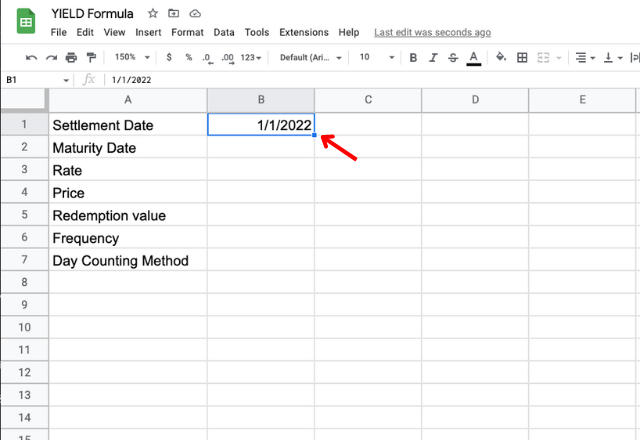#### 2. Maturity date

Now that you have filled in the cell with the settlement date, let’s move on to the next step.

The maturity date is the date on which the seller must repay the principal amount to the investor. In other words, this is the day when the debt, or the investment, reaches its end.

Again, it’s important to enter the date in a valid format.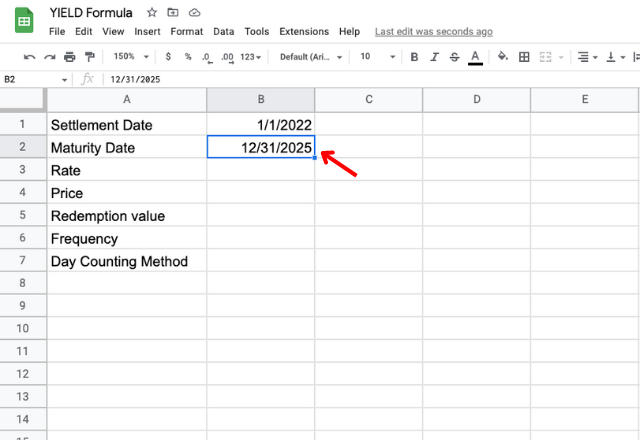#### 3. Rate

This is the rate at which the investment appreciates. The rate is expressed as a percentage over the principal amount. Let’s say a bond pays an interest rate of 4.2%. In this case, type in 4.2%.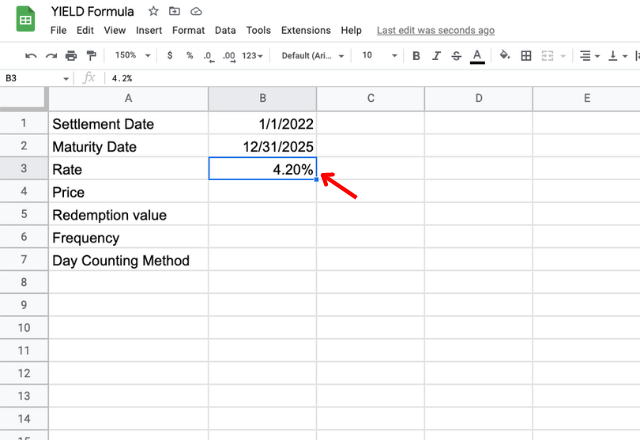#### 4. Price

The price is the value at which the stock or bond is traded. Make sure you enter the price in a valid format.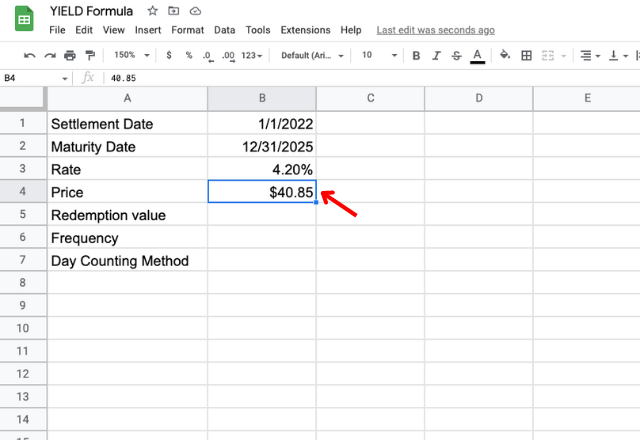#### 5. Redemption

When it comes to finance, the redemption occurs when the investor sells the stocks or the bonds back to the company. Thus, the redemption value is the current market price at which the security is traded.

Now enter the redemption value in a valid format.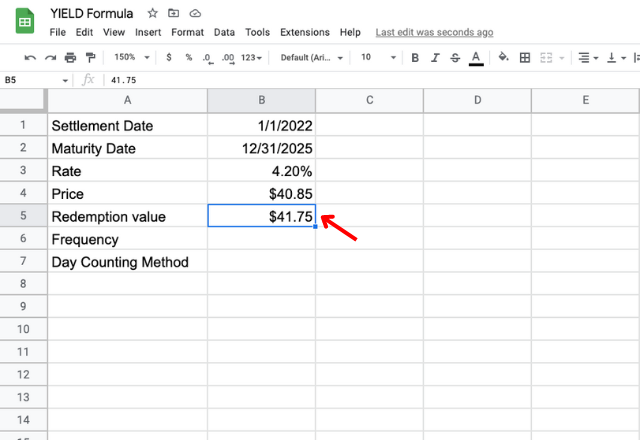#### 6. Frequency

This is related to the frequency in which you receive dividends or the interest rate over the principal. For example, stocks can pay dividends quarterly, semiannually or annually.

In the YIELD formula, the frequency is the number of payments per year. Therefore, enter number 1 (annually), 2 (semiannually) or 4 (quarterly).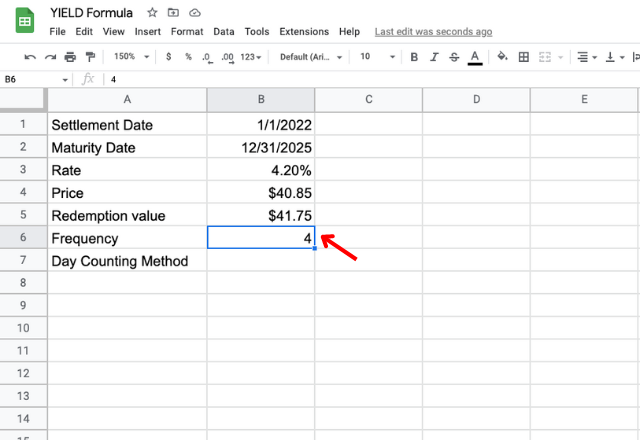#### 7. Day count

A day count convention is a standardized system that determines how interest accrues over time. The most commonly used convention is the 30/360, which considers all months to have 30 days in length and each year to have 360 days.

If that’s the case, type in 0.

Note: This is the default value in the YIELD formula. If you choose not to type in any number, Google Sheets will consider the 30/360 day count convention by default.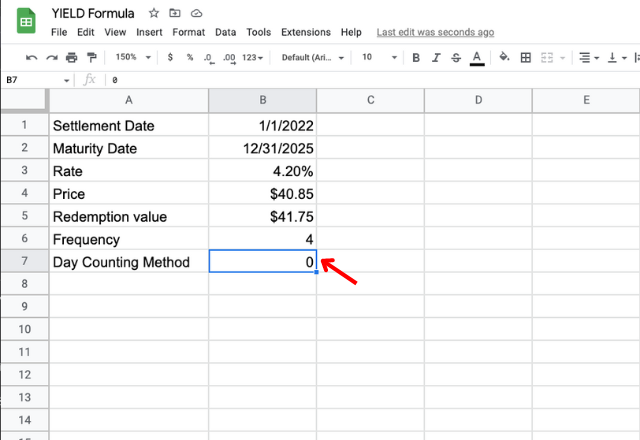#### 8. YIELD formula

Now that you have all the variables all you need to do is apply the YIELD formula in Google Sheets.

Type in the formula and add the corresponding cells as variables.

=yield(B1,B2,B3,B4,B5,B6,B7)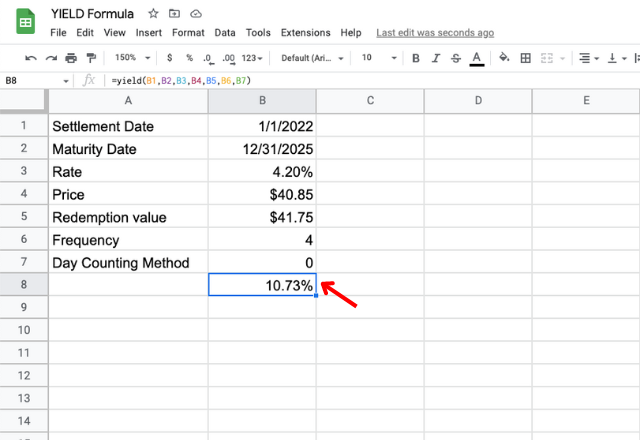Another option is to type in the YIELD formula with all the corresponding variables between parentheses.

=yield(“1/1/2022″,”12/31/2025”,4.20%,40.85,41.75,4,0)

Note: In this case, don’t forget to use commas to separate the variables and to include quotation marks around the dates when inputting the YIELD formula.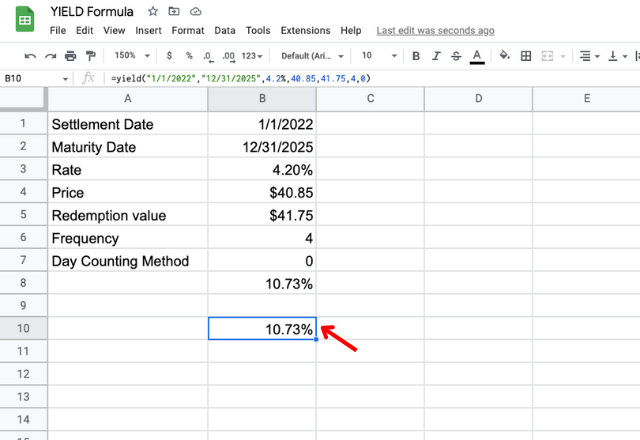## How to use the YIELD formula in Google Sheets

So there you have it! You have just learned how to use the YIELD formula in Google Sheets.

If you want to learn some other useful financial functions, check out this article on how to use the FV formula in Google Sheets.